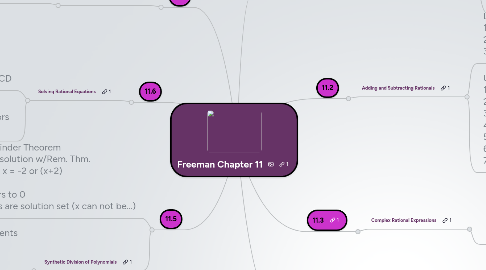# Freeman Chapter 11

Get Started. It's FreeFreeman Chapter 11## 1. 11.3

### 1.1. Complex Rational Expressions

1.1.1. GOALS: LCD 1. Add fractions 2. Get rid of fractions

1.1.1.1. 1. *Factor denominator 2. Find LCD 3. Multiply every fraction by LCD/1 to rid fractions 4. In single fraction now - check for negative distribution 5. Factor or combine like terms or reduce fractions

## 2. 11.1

### 2.1. Rationals: Multiply and Divide & Graphs

2.1.1. Simplifying algebraic fractions *reminders: 1. Opposites = -1 and cancel = 1 2. Always check for cross cancel 3. Reduce answers in factored form

## 3. 11.4

### 3.1. Long Division of Polynomials

3.1.1. Long division of polynomials 1. Using red pencil helps maintain proper negative and positive numbers

## 4. 11.2

### 4.1. Adding and Subtracting Rationals

4.1.1. Like Denominators 1. Add numerators 2. Keep denominators 3. Factor and reduce

4.1.2. Unlike Denominators 1. Factor denominators 2. Find LCD 3. Write new LCD 4. *Look for whats missing 5. Simplify numerator 6. Add or subtract numerator 7. Simply and circle

## 5. 11.5

### 5.2. Synthetic Division of Polynomials

5.2.1. 1. Order by powers using 0x for place-marking missing powers, and write coefficients 2. Set divisor = 0 (root) 3. Write "sort of a backwards division sign" and bring down 1st coefficient 4. Multiply root by 1st coefficient and add 2nd coefficient 5. Repeat step 4 until done 6. Reorder backwards from remainder

## 6. 11.6

### 6.1. Solving Rational Equations

6.1.1. With = Sign 1. Variable in Denominator 2. Wipe out fractions with LCD

6.1.1.1. Check for "answers can not ="

6.1.2. No = Sign 1. Add or subtract with common denominators 2. Factor, simplify and double check

## 7. 11.7

### 7.1. Formulas and Applications

7.1.1. 1. Determine if problem is work or motion

7.1.1.1. Work 1. Rate / Time / Part of Job 2. 1/12 / x / x/12 3. 1 job/time + 1 job/time = 1/1

7.1.1.2. Motion 1. Rate x Times = Distance 2. Determine What your solving for, rate, time, or distance 3. Solve for x (are times = or are they addition??)